Back to Table of Contents [TOP]  Combining the first and second laws of thermodynamics - The fundamental equations of thermodynamics (1) The fundamental equations of thermodynamics The first law of thermodynamics may be written as:where for a reversible change a closed system of constant composition, and in the absence of non-expansion work we have:Therefore we may re-write the first law as: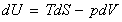THIS REALTIONSHIP IS KNOWN AS THE FUNDAMENTAL EQUATION OF THERMODYNAMICS .  We will revisit this equation at a later stage to include the effects of changing the composition of the system (the fundumental equation of chemical thermodynamics.) This equation shows how the internal energy of a closed system changes with changes in S and V, i.e. U = U(S,V). Now, because dU is an exact differential (U is a state function), its value is path independent, i.e. this equation is valid for all types of processes of a closed system that does no non p-V work.      (2) Use of the Fundamental Equation to generate new functions (Derivations not examinable.) If we where to differentiate U = U(S,V) we get:i.e. from the fundamental equation we see that:and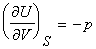Another very interesting equation (one of a set called the Maxwell relationships) may be obtained from the property that if for U = U(x,y) , dU is an exact differential, then we have: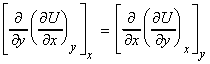which in our case we have:i.e.:or: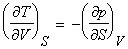NOTE: The full set of the Maxwell relationships may be derived form the other thermodynamic functions and are summarised as follows (not examinable):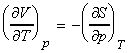CH237 - Chemical Thermodynamics and Kinetics

 BACK TO TABLE OF CONTENTS   Combining the first and second laws of thermodynamics - The fundamental equations of thermodynamics (1) The fundamental equations of thermodynamics The first law of thermodynamics may be written as:where for a reversible change a closed system of constant composition, and in the absence of non-expansion work we have:Therefore we may re-write the first law as:THIS REALTIONSHIP IS KNOWN AS THE FUNDAMENTAL EQUATION OF THERMODYNAMICS . It shows how the internal energy of a closed system changes with changes in S and V, i.e. U = U(S,V). Now, because dU is an exact differential (U is a state function), its value is path independent, i.e. this equation is valid for all types of processes of a closed system that does no non p-V work.  (2) Use of the Fundamental Equation to generate new functions  If we where to differentiate U = U(S,V) we get:i.e. from the fundamental equation we see that:andAnother very interesting equation (one of a set called the Maxwell relationships) may be obtained from the property that if for U = U(x,y) , dU is an exact differential, then we have:which in our case we have:i.e.:or:NOTE: The full set of the Maxwell relationships may be derived form the other thermodynamic functions and are summarised as follows (not examinable):[ UNIVERSITY OF MALTA | FACULTY OF SCIENCE | DEPARTMENT OF CHEMISTRY ]

 E-mail me at jgri1@um.edu.mt http://staff.um.edu.mt/jgri1